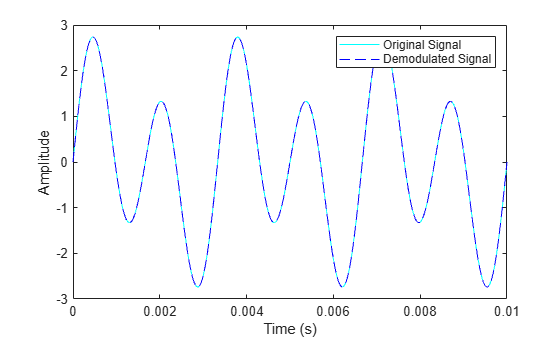Documentation

This is machine translation

Mouseover text to see original. Click the button below to return to the English version of the page.

amdemod

Amplitude demodulation

Syntax

```z = amdemod(y,Fc,Fs) z = amdemod(y,Fc,Fs,ini_phase) z = amdemod(y,Fc,Fs,ini_phase,carramp) z = amdemod(y,Fc,Fs,ini_phase,carramp,num,den) ```

Description

`z = amdemod(y,Fc,Fs)` demodulates the amplitude modulated signal `y` from a carrier signal with frequency `Fc` (Hz). The carrier signal and `y` have sample frequency `Fs` (Hz). The modulated signal `y` has zero initial phase and zero carrier amplitude, so it represents suppressed carrier modulation. The demodulation process uses the lowpass filter specified by ```[num,den] = butter(5,Fc*2/Fs)```.

Note

The `Fc` and `Fs` arguments must satisfy `Fs` > 2(`Fc` + `BW`), where `BW` is the bandwidth of the original signal that was modulated.

`z = amdemod(y,Fc,Fs,ini_phase)` specifies the initial phase of the modulated signal in radians.

`z = amdemod(y,Fc,Fs,ini_phase,carramp)` demodulates a signal that was created via transmitted carrier modulation instead of suppressed carrier modulation. `carramp` is the carrier amplitude of the modulated signal.

`z = amdemod(y,Fc,Fs,ini_phase,carramp,num,den)` specifies the numerator and denominator of the lowpass filter used in the demodulation.

Examples

collapse all

Set the sample rate and carrier frequency.

```fc = 10e3; fs = 80e3;```

Generate a sinusoidal signal having a 0.01 s duration.

```t = [0:1/fs:0.01]'; s = sin(2*pi*300*t)+2*sin(2*pi*600*t);```

Create a lowpass filter.

`[num,den] = butter(10,fc*2/fs);`

Amplitude modulate the signal, `s`.

`y = ammod(s,fc,fs);`

`s1 = amdemod(y,fc,fs,0,0,num,den);`

Plot the original and demodulated signals.

```plot(t,s,'c',t,s1,'b--') legend('Original Signal','Demodulated Signal') xlabel('Time (s)') ylabel('Amplitude')```The demodulated signal is nearly identical to the original signal.